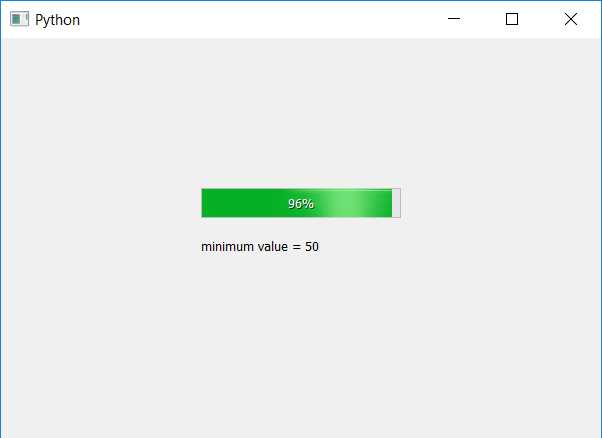# PyQt5 – minimum() method for Progress Bar

• Last Updated : 22 Apr, 2020

Progress Bar is a widget that is used when we process lengthy tasks. It is animated so that the user knows that the task is progressing, it is basically graphical control element used to visualize the progression of an extended computer operation, such as a download, file transfer, or installation.

We can set the minimum value of progress bar using `setMinimum` method, `minimum` method is used to get the minimum value of desired progress bar.

Syntax : bar.minimum()

Argument : It takes no argument.

Return : It returns integer which refers the minimum value of progress bar.

Code :

 `# importing libraries``from` `PyQt5.QtWidgets ``import` `*` `from` `PyQt5 ``import` `QtCore, QtGui``from` `PyQt5.QtGui ``import` `*` `from` `PyQt5.QtCore ``import` `*` `import` `sys`` ` ` ` `class` `Window(QMainWindow):`` ` ` ` `    ``def` `__init__(``self``):``        ``super``().__init__()`` ` `        ``# setting title``        ``self``.setWindowTitle(``"Python "``)`` ` `        ``# setting geometry``        ``self``.setGeometry(``100``, ``100``, ``600``, ``400``)`` ` `        ``# calling method``        ``self``.UiComponents()`` ` `        ``# showing all the widgets``        ``self``.show()`` ` `    ``# method for widgets``    ``def` `UiComponents(``self``):`` ` `        ``# creating progress bar``        ``bar ``=` `QProgressBar(``self``)`` ` `        ``# setting geometry to progress bar``        ``bar.setGeometry(``200``, ``150``, ``200``, ``30``)`` ` `        ``# setting minimum value of progress bar to 50``        ``bar.setMinimum(``50``)`` ` `        ``# setting value to progress bar``        ``bar.setValue(``98``)`` ` `        ``# getting minimum value of progress bar``        ``value ``=` `bar.minimum()`` ` `        ``# creating label to print the value``        ``label ``=` `QLabel(``"minimum value = "` `+` `str``(value), ``self``)`` ` `        ``# adjusting the size of label``        ``label.adjustSize()`` ` `        ``# moving the label``        ``label.move(``200``, ``200``)`` ` ` ` ` ` `        ``# setting alignment to centre``        ``bar.setAlignment(Qt.AlignCenter)`` ` ` ` `# create pyqt5 app``App ``=` `QApplication(sys.argv)`` ` `# create the instance of our Window``window ``=` `Window()`` ` `# start the app``sys.exit(App.``exec``())`

Output :My Personal Notes arrow_drop_up